Published: 01 August 2023

# Optimization of communication performance in wireless seismic monitoring system

Yonghong Fan1
Daoping Han2
Na Li3
1, 2, 3Qingdao Huanghai University, Qingdao, China
Corresponding Author:
Yonghong Fan
Views 29

#### Abstract

In order to improve the communication performance between different equipment in the seismic detection system, a wireless network system with MIMO (Multiple Input and Multiple Output) characteristics was designed and applied in the paper. On the basis of 5G communication, a new data conversion and transmission model was built, which can improve the rate of signal transmission and reduce the bit error rate and power consumption. For the purpose of power optimization, the overall framework of the network system was improved, of which power characteristics and performance were verified. The conjugate beam was normalized, and the cumulative distribution function under different downlink rates was obtained based on the maximum minimum power control scheme. In order to verify the energy-saving effect of the design, the ADC (Analog-to-Digital Conversion) power model is introduced. Through simulation analysis, the change rules of spectrum efficiency and energy efficiency under different network nodes were obtained. The results show that the normalized conjugate beam can be less affected by the transmission nodes, and the power control is more reliable under the same conditions. The research and application of this subject can significantly improve the wireless communication performance of the seismic monitoring system, reduce the power consumption of network equipment, improve the data transmission efficiency, and provide strong technical support for seismic prediction and data analysis. Through the test of artificial earthquake, it is verified that the communication scheme designed in this paper has higher transmission efficiency and lower bit error rate, and is very suitable for the long-distance transmission of seismic signals.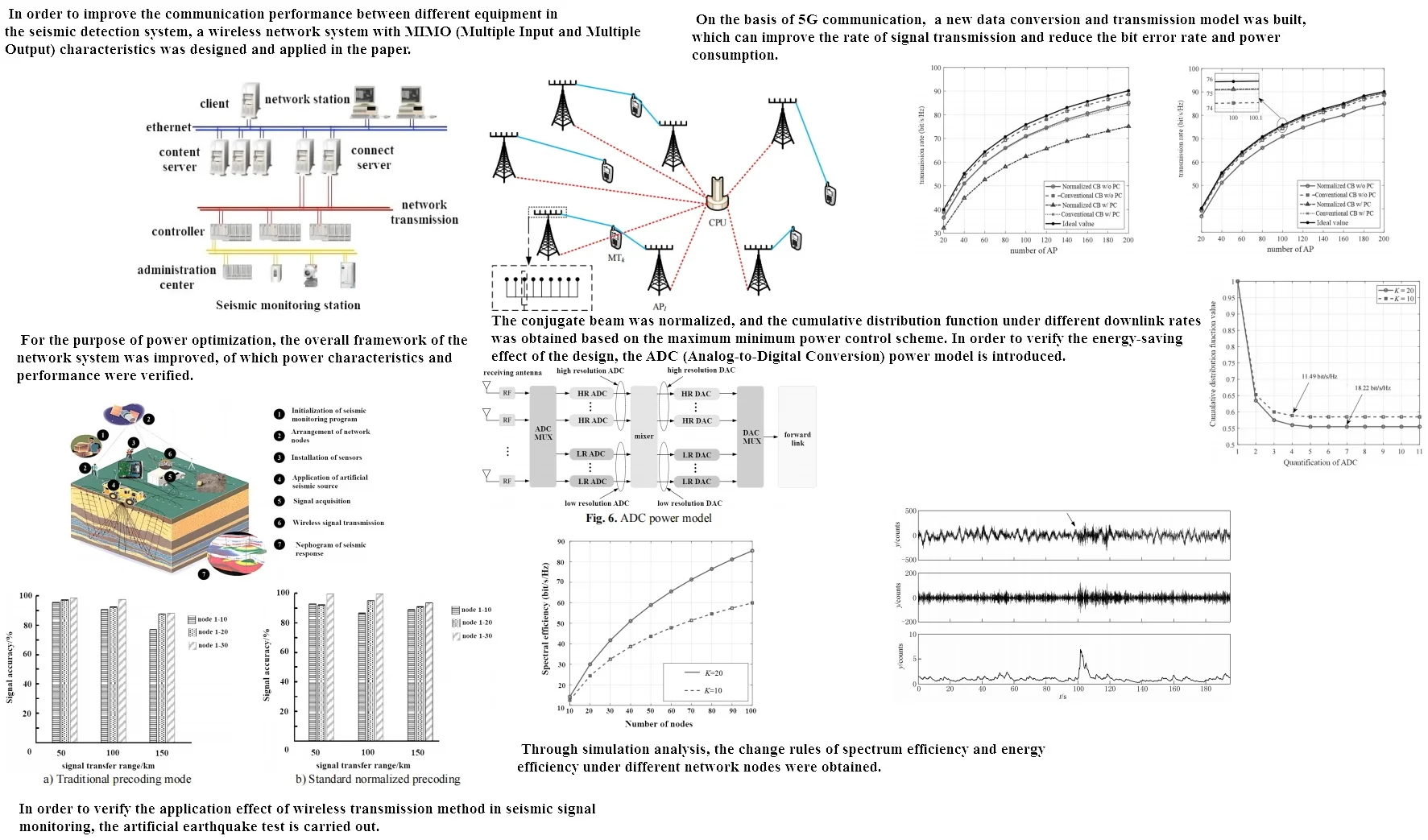#### Highlights

• On the basis of 5G communication, a new data conversion and transmission model was built, which can improve the rate of signal transmission and reduce the bit error rate and power consumption.
• The conjugate beam was normalized, and the cumulative distribution function under different downlink rates was obtained based on the maximum minimum power control scheme.
• Through simulation analysis, the change rules of spectrum efficiency and energy efficiency under different network nodes were obtained.

## 1. Introduction

Seismic disasters are characterized by suddenness and strong destructive force, which will damage traffic facilities such as roads and railways, even villages and towns, and cause great harm to life and property. Therefore, cities need to set up seismic detection platforms. At present, most of the seismic monitoring sensors are widely set up in harsh traffic environments such as suburbs, resulting in insufficient efficiency of on-site data acquisition, greatly reduced maintenance efficiency of station observation data acquisition equipment, and unable to process data information timely, quickly and accurately. In order to improve the information level of the seismic detection system and ensure the timeliness and accuracy of seismic data, it is very important to upload the acquired data to the central monitoring terminal in real time. With the support of wireless network and communication technology, seismic data interaction and centralized control between different cities or regions can be realized. In order to improve the identification ability of vibration signals, it can be combined with neural network technology to facilitate the extraction of parameter modes . In terms of seismic signal transmission, there is a big gap between 4G and 5G modes, including the number and scale of base stations. Therefore, the application of a wireless network system based on 5G transmission mode in seismic testing is proposed and verified in this paper. With the increase of the number of detection terminals, this brings higher performance requirements to the wireless network system. For example, seismic data transmission in remote or remote areas is efficient and uninterrupted, and it is difficult to expand more network equipment without affecting the network speed . In addition, the surrounding high-power and high radiation equipment have strong interference to the network. Therefore, the stability and power control of the network become the key problems to be solved in the communication system. At present, boundary interference is one of the key factors affecting the reliability of wireless communication in seismic monitoring system. When the sensor is located at the edge of the network, the probability of signal distortion is relatively high. Since the application and development of network technology in industrial production is transitional , the corresponding data transmission standard should be replaceable , which is also the premise of performance optimization. In order to solve these problems, a wireless network optimization design scheme of seismic monitoring system is proposed in this paper. The conjugate beam  is standardized, and its power control effect and its influence on the spectral efficiency and energy efficiency of the system are studied. At the same time, in order to reduce the cost of the network architecture, a composite A/D conversion power model  is introduced to achieve good cost performance. In addition, the system takes mobile terminals as the center to design the network structure, which can effectively establish the data connection with the base station, reduce boundary interference, and play a positive role in promoting the development of intelligent seismic engineering. In Chapter 2, the wireless network transmission structure and power control model of seismic signals are constructed, and the changes of short-term power control and long-term power are obtained. In Chapter 3, the performance parameters of the designed system are calculated and verified, including spectrum efficiency analysis and energy efficiency analysis.

## 2. Network structure design of seismic monitoring system

### 2.1. Overall frame design

The network communication process of the seismic monitoring system is shown in Fig. 1. It can be seen that the number and layout of monitoring stations are determined according to the regional characteristics. The layout, installation, data transmission and control of all communication equipment are carried out in the monitoring station, including the management center and the monitoring center. For long-distance seismic data transmission, wireless network system preferentially adopts non cellular large-scale MIMO structure, as shown in Fig. 2, which greatly reduces the complexity of deployment site and is suitable for weak signal environment. At the same time, the decentralized base stations can completely cover the mobile terminals, and improve the macro diversity efficiency on the premise of ensuring the communication quality. Although the number and distribution density of communication nodes can be adjusted according to the actual situation, there is still a certain amount of interference and superposition of base station signals in different coverage areas .

Fig. 1Communication process of the system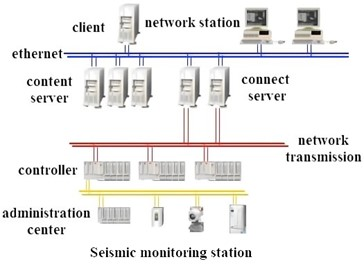Fig. 2Structure of wireless network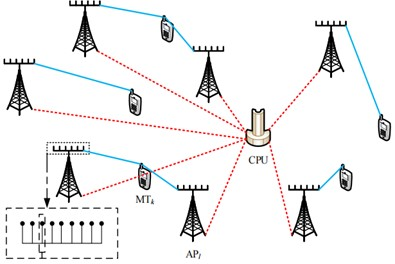In order to accurately peel off the effective signals of seismic data, the multi-point joint transmission technology  can be introduced into the system, that is, the distributed control strategy is used to classify and cooperate with each base station, establish a reasonable communication connection combination, and finally achieve the effect of reducing the impact of interference signals. In addition, with the development and application of optical fiber communication technology  and network cooperation algorithm , the full function controller can flexibly control the transmission power, which has a good effect in energy saving and consumption reduction. In order to verify the advantages of this communication structure, it is necessary to analyze its frequency efficiency and energy efficiency.

### 2.2. Data transmission model

In the seismic monitoring system, it is assumed that there are $N$ power increasing antennas in the data transmission system. Among them, there are $M$ antennas in the same operating period and $K$ antennas in the same frequency band, randomly distributed in a given communication area. When the wireless network access points in the system return data, they need to use the CPU unit to ensure the consistency of the network information type and reasonably control the load data and power coefficient. The data transmission from the network node to the mobile terminal mainly includes two parts: uplink training and downlink transmission control. Since the terminal data needs a pilot sequence, precoding should be performed in advance in the downlink .

Let ${g}_{mk}$ be the channel coefficient between the $k$th user and the $m$th network node, then ${g}_{mk}$ in the downlink is expressed as:

1
${g}_{mk}=\sqrt{{\beta }_{mk}}{h}_{mk},$

where ${\beta }_{mk}$ is a large-scale fading coefficient, and its path loss and shadow effect need to be considered. The value is independent of the antenna index. ${H}_{mk}$ is a small-scale fading coefficient, which is independent in a specific time period and belongs to a random variable.

In the wireless communication modular, the coherence interval length of data transmission is set to be $T$, which is slightly larger than the uplink training duration sample ${\tau }_{up}$, then the pilot vector ${y}_{m,up}$ accepted by the $m$th node can be expressed as:

2
${y}_{m,up}=\sqrt{{\tau }_{up}{\rho }_{up}}\sum _{k=1}^{K}{g}_{mk}{\varphi }_{k}+{w}_{m,up},$

where ${\rho }_{up}$ is the signal-to-noise ratio of signal transmission, which is related to the pilot sequence and has normalization characteristics; ${w}_{m,up}$ is the pilot sequence vector, which belongs to additive Gaussian white noise vector. After the pilot transmission is completed, the $m$th network node will obtain the inner product of the pilot vector and the signal-to-noise ratio vector to realize uplink training.

In the downlink data transmission stage, the signal used for data communication shall be based on the user’s terminal information. Under the normalization condition, the data transmitted by the $m$th node can be expressed as:

3
${x}_{m}=\sqrt{{\rho }_{d}}\sum _{k=1}^{K}\sqrt{{\gamma }_{mk}}\frac{{{\stackrel{^}{g}}^{\mathrm{*}}}_{mk}}{\left|{\stackrel{^}{g}}_{mk}\right|}{s}_{k},$

where ${s}_{k}$ is the signal that the system expects to send to the $k$th terminal, ${\rho }_{d}$ is the signal transmission power coefficient of the network node, which can effectively control and limit the peak power. ${\gamma }_{mk}$ represents the power control coefficient.

Compared with the conventional conjugate beam, the corresponding long-term power constraint equation can be expressed as:

4
$\sum _{k=1}^{K}{\mu }_{mk}{\alpha }_{mk}\le 1,\mathrm{}\mathrm{}\mathrm{}\mathrm{}\mathrm{}m=1,\dots ,M,$

where ${\alpha }_{mk}$ is the substitution quantity, and its expression is:

5
${\alpha }_{mk}\triangleq \frac{{\tau }_{up}{\rho }_{up}{{\beta }^{2}}_{mk}}{{\tau }_{up}{\rho }_{up}{\beta }_{mk}+1}.$

According to the data transmission model, with the increase of the number of network nodes, the signal interference and noise are significantly weakened. This conclusion is consistent with the downlink situation using the normalized precoder.

### 2.3. Optimal power control analysis

According to the characteristics of the data transmission model, the actual transmission power of each network node is affected by ${\rho }_{d}$ is significant, especially for short-term power control. The maximum and minimum power constraint equations can be expressed as:

6
$\underset{\left\{{\eta }_{mk}\right\}}{\mathrm{m}\mathrm{a}\mathrm{x}}\mathrm{}\mathrm{}\underset{k=1,...,K}{\mathrm{m}\mathrm{i}\mathrm{n}}\mathrm{}{R}_{d,k}^{st},$
$\begin{array}{ccc}\mathrm{s}\mathrm{u}\mathrm{b}\mathrm{j}\mathrm{e}\mathrm{c}\mathrm{t}\mathrm{}\mathrm{t}\mathrm{o}& \sum _{k=1}^{K}{\gamma }_{mk}\le 1,& m\mathrm{}=\mathrm{}1,\mathrm{}.\mathrm{}.\mathrm{}.,\mathrm{}M,\\ & {\gamma }_{mk}\ge 0,& k\mathrm{}=\mathrm{}1,\mathrm{}\dots ,\mathrm{}K,\mathrm{}\mathrm{}\mathrm{}\mathrm{}\mathrm{}m\mathrm{}=\mathrm{}1,\mathrm{}\dots ,\mathrm{}M.\end{array}$

Under the constraint condition, solving the extreme value of the equation is the key of power optimization control. In order to facilitate the solution of the equation, the cumulative distribution function values under different downlink rates  are analyzed based on the conjugate beam normalization and balanced power control method  to determine the power control effect. In the local area, the number of power increasing antennas in the same working period is set to 60, and the number of the same frequency band is set to 20. By comparing and analyzing the conditions of normalized CB and conventional CB, the characteristic curves of short-term power control and long-term power control are shown in Fig. 3 and Fig. 4 respectively. It can be seen that in terms of power control, the standardized conjugate beam precoding is obviously superior to the traditional scheme; From the specific numerical analysis, the link rate of the standardized conjugate beam precoder is 2.07 bit/s/Hz under the condition of less than 5 % interruption, which is 1.15 times higher than the normal condition. This is because the CB precoder after normalization and normalization processing can provide greater gain and maximize the minimum rate of data transmission. As the work cycle of different seismic monitoring base stations varies greatly, wireless network communication equipment is selected according to the actual situation to obtain better work effect on the premise of ensuring the basic performance, compatibility and matching.

Through the comparison and analysis of no power control curve, it can be seen that power control is one of the effective methods to improve the link rate, which can reduce the interruption rate by more than 12 %. Under the condition of low signal-to-noise interference ratio, the coherent beam  forms a significant gain. For example, the adaptive antenna system can improve the signal-to-noise ratio by about 40 dB under the strong interference environment. Under the condition control based on the maximum minimum power distribution standard, the performance of CB precoder is less affected by the number of terminals, so there will be no efficiency interference between different terminals. Under the control of the maximum minimum power distribution standard, the cumulative distribution function change law under different node numbers can be obtained, as shown in Fig. 5. It can be seen that the power allocation scheme can maintain the stability of network nodes under different coding conditions; The standardized conjugate beam precoding consumes less power under the same number of network nodes and is suitable for network nodes with significant mobility.

Fig. 3Short term power control characteristic curve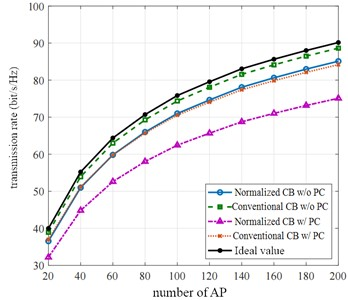Fig. 4Long term power control characteristic curve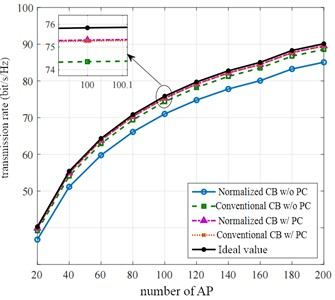Fig. 5Cumulative distribution function value under different node numbers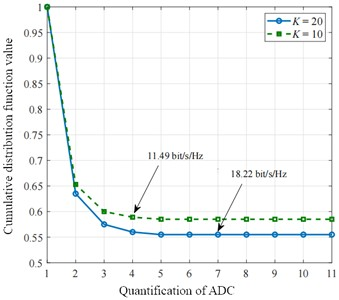## 3. Power consumption performance analysis of seismic monitoring system

### 3.1. Spectrum efficiency analysis

In order to avoid the influence of signal interaction, the signals sent by different terminal antennas have no correlation, including the quantized Gaussian noise. In order to further improve the spectral efficiency and energy efficiency of the system, a digital to analog conversion (ADC) power model is introduced into the added term, as shown in Fig. 6, which can effectively suppress the influence of noise. The functions of uplink and downlink in the network system are different. The uplink is mainly used to transmit pilot sequences to network nodes and complete channel estimation, which has an important impact on the standardized conjugate beam precoding of the downlink. Spectral efficiency is one of the important parameters to measure uplink performance. Especially under the condition of low resolution ADC, it can effectively determine the power loss. In addition, since the spectral efficiency is interfered by the production equipment, the loss coefficient is selected according to the effective number of equipment during calculation.

In order to study the influence of the number of network nodes on the spectral efficiency, the spectral efficiency change is calculated under the condition that the number of network nodes $K$ in the same frequency band is 20 and 10 respectively. The results are shown in Fig. 7. It can be seen that with the increase of the number of network nodes working in the same period, the spectrum efficiency of the system increases obviously, but it gradually presents a temporary phenomenon; The more nodes in the same frequency band, the greater the spectrum efficiency. For the seismic monitoring system, the number of network nodes needs a reasonable selection range, otherwise it is difficult to promote the reduction of power consumption. If the communication distance between different seismic monitoring base stations is short, the number of nodes should be appropriately reduced to optimize the spectral efficiency with power consumption as the index. Spectral efficiency is one of the indicators to measure the anti-interference ability of communication systems, and its variation rule is an important basis for selecting relevant auxiliary equipment.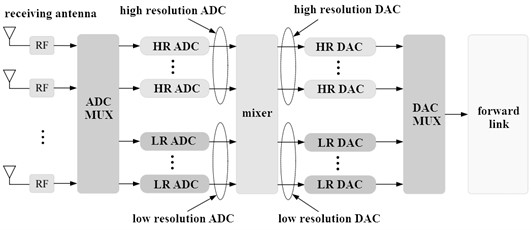Fig. 7Spectrum efficiency under different number of nodes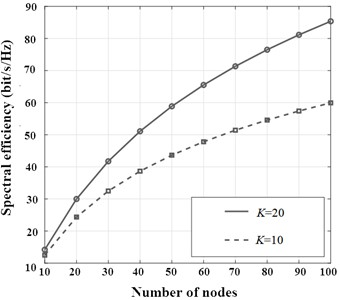The comprehensive use of high-resolution and low-resolution ADCs can effectively reduce power consumption and improve the performance price ratio of the system. However, with the decrease of ADC resolution, the performance loss is more significant, which is manifested as the decrease of transmission rate and spectral efficiency. If this problem occurs, the power increasing antenna can be added within a certain range to make up for the distortion of the signal and meet the optimization design requirements of the system.

### 3.2. Energy efficiency analysis

In the square area of 100,000 m2, assuming that the network nodes and mobile terminals of each base station are randomly distributed, the energy efficiency is calculated by the surrounding principle, and the energy efficiency change law under different node numbers can be obtained as shown in Fig. 8. It can be seen that with the increase of the number of network nodes in the same period, the energy efficiency changes in a decaying trend; Similar to the change of spectrum efficiency, the more nodes in the same frequency band, the higher the energy efficiency.

Fig. 8Energy efficiency under different node numbers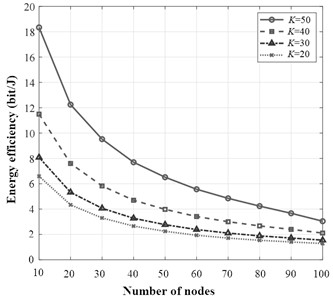Since the ADC power model is introduced into the system, the number of quantization bits will have a certain impact on the energy efficiency. Under the condition of different number of network nodes in the same frequency band, the change law of energy efficiency with the number of quantization bits of digital analog / digital conversion can be obtained, as shown in Fig. 9. It can be seen that when the number of network nodes $K$ in the same frequency band is less than 30, the energy efficiency basically does not change; With the increase of $K$ value, the energy efficiency shows a trend of increasing first and then decreasing. When the number of quantization bits is 4, the energy efficiency reaches an extreme value. The allocation and arrangement of network nodes have a key impact on the spectrum efficiency and energy efficiency. In the case of random distribution, the energy efficiency of a single area does not increase with the increase of the number of network nodes, because the average effective transmission distance in the service area increases.

Fig. 9Energy efficiency under different quantization bits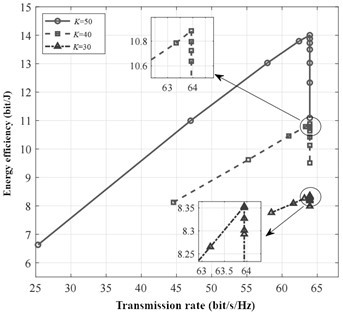### 3.3. Influence of channel on propagation rate

The spectrum efficiency under different channels are shown in Fig. 10. It can be seen that due to the constraint of Shannon’s theorem, the growth rate under different channels gradually slows down. In addition, the system has a higher sum rate in rice channel than in Rayleigh channel. Rice channel suffers less signal attenuation than Rayleigh channel, which is consistent with the expected result of theoretical analysis. When the low resolution ADC is considered, the influence of the quantization distortion on the uplink performance is reflected in the channel estimation and signal detection stages. And the impact on downlink performance is only reflected in the channel estimation stage. On the other hand, the downlink itself enjoys a larger transmission power than the uplink. This indicates that it is recommended to use an ADC at the AP side of a large-scale MIMO system in cellular manner to reduce deployment cost and power consumption, while maintaining the same system and rate as in the case of a perfect ADC.

Fig. 10Spectrum efficiency under different channels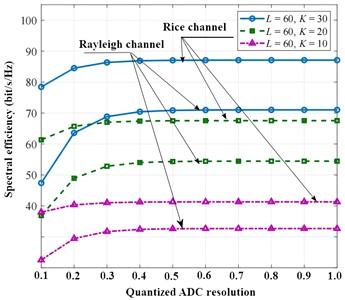## 4. Analysis of seismic monitoring effect

### 4.1. Test principle and scheme of artificial earthquake

In order to verify the application effect of wireless transmission method in seismic signal monitoring, the artificial earthquake test is carried out within 106 m2. The principle of artificial seismic test is shown in Fig. 11. The formation of artificial earthquake depends on the impact load of seismic vehicle. The four hydraulic shafts in the middle of the wheel are connected to the seismic hammer located at the lower part of the vehicle body. The hydraulic shafts lift the seismic hammer up and down through telescoping and then hit the ground. A strong force impacts the underground rock stratum, and an artificial earthquake is formed. The seismic vehicle will have excitation vibration every other distance, and the seismic signal detection, filtering and amplitude quantization processing can be obtained as shown in Fig. 12. Only in this way can the geophone at the artificial seismic site accurately monitor the best response of the underground strata.

Fig. 11Test principle of artificial earthquake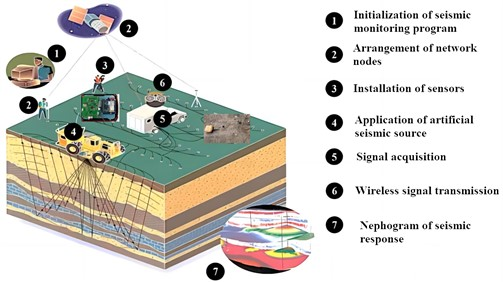The seismic signal test of the wireless transmission system mainly includes four aspects, such as the test of the connection status between the upper computer program and the data acquisition equipment, the test of the node seismograph data acquisition and transmission parameters, the test of the status information of the seismograph equipment and the test of the node seismograph data file transmission efficiency. According to the characteristics of the channel, the energy or amplitude of the signal is taken as the characteristic function. For each measuring point, the seismograph node used in the experiment should ensure good initialization effect to achieve ideal data transmission and stable flow rate. According to the verification of the nodes, the seismic data transmission system has been in normal working condition during the whole vibration excitation period, without transmission interruption. It can be seen that the wireless network structure built in this experiment has a good coverage, and the seismic data transmission system can meet the verification of data transmission effect in this range.

Fig. 12Seismic signal detection, filtering and amplitude quantization processing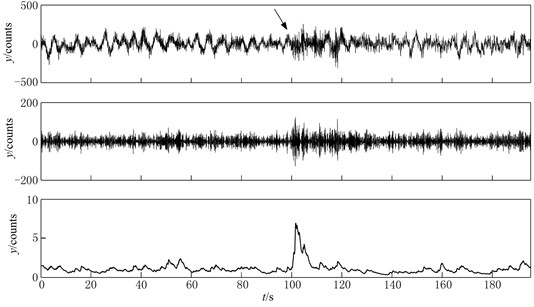Fig. 13General layout of seismic survey points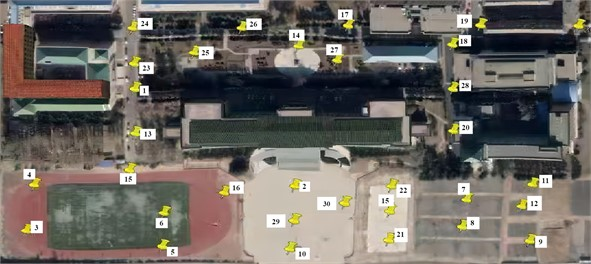In the seismic monitoring test, the layout of seismograph nodes should meet the requirement that the travel difference of seismic signals from the deepest source to the nearest and farthest seismograph nodes should reach at least one wavelength. Therefore, according to this theory, this experiment deploys seismograph nodes from the fracturing center at a distance of approximately 100 m within the fracturing range of 1.5 kilometers, and arranges 30 seismograph nodes as shown in Fig. 13. After the seismograph node array is deployed, a wireless relay network needs to be built on site to cover the entire vibration area. According to the characteristics of the star array structure, the communication distance of the wireless communication module and the maximum bandwidth. This experiment uses two master relay nodes and three slave relay nodes to form three direct links. Two master relay nodes are arranged near the control center, and the two master relay nodes are connected to the control center in a wired way. The three slave relay nodes are respectively arranged on the bisector between the two survey lines of the well pad, mainly to reduce and balance the load. Set the receiving terminal of seismic signal to be about 100 kilometers away from the center of the test range. Through the test of data stream transmission efficiency, throughput and delay, the impact of different transmission methods and coding types on wireless transmission performance can be compared and verified. In the computational time domain, the first arrival time of microseism is recorded by the extreme value of the ratio of two time windows. However, before the arrival of the first arrival of the micro-earthquake, there is only background noise in the record. After the arrival of the first arrival, the effective signal is mixed with noise, and the energy mutation point before and after the first arrival is recorded as the first arrival time of the signal.

### 4.2. Test of seismic signal transmission efficiency

The control function of the upper computer program on the seismograph equipment of each node is more specific, which is mainly manifested by sending information to a designated node seismograph equipment through the convergence center node, and then taking the packet return rate and network delay as indicators, that is, the transmission rate of information. In order to realize the transmission rate test of wireless network transmission system, this paper selects the node seismograph equipment with the largest signal strength as the convergence point, and sends information to all node seismograph equipment through the upper computer program. As the number of nodes increases, the distance between the corresponding seismograph equipment and the convergence point increases, the packet return rate in the transmission rate index decreases, and the network delay increases. However, at this time, all nodes connected to the network can respond to the information sent by the upper computer program in a timely manner. Through the status information query, the status information of the current node seismograph equipment and the adjacent equipment can be displayed, and the parameters of the corresponding modified node seismograph equipment can also be displayed normally by randomly modifying some equipment configuration parameters. In addition, the transmission rate performance test of reflecting node seismograph equipment is the inter-hop transmission capacity test. The test method is to obtain the time interval of return of status information after sending information instructions to the farthest node through the upper computer program of the central node. The transfer capacity of inter-hop is mainly determined by two variables. The first is the average rate of inter-hop, which is calculated by dividing the total amount of data transmitted by the network access node seismograph equipment by the completion time. The other is the inter-hop network throughput, which is calculated by dividing the total network throughput by the transmission time. The signal receiving groups of seismograph measuring points are set to 10, 20 and 30 respectively, which can effectively verify the effect of the number of nodes on the communication quality.

Table 1Performance parameters in non cellular large-scale MIMO structure mode

 Number of nodes Average data stream transmission efficiency / kbps Average throughput / Mbps Average delay amount / μs 1-10 ($K=$10) 3986 11.6 320 1-20 ($K=$20) 5382 12.7 415 1-30 ($K=$30) 6387 14.6 625

Table 2Performance parameters in traditional parallel communication mode

 Number of nodes Average data stream transmission efficiency / kbps Average throughput / Mbps Average delay amount / μs 1-10 ($K=$10) 2376 18.3 525 1-20 ($K=$20) 4896 21.7 730 1-30 ($K=$30) 5336 24.2 990

For seismograph nodes, the transmission performance parameters are obtained in non cellular large-scale MIMO structure and traditional parallel communication mode, as shown in Table 1 and Table 2 respectively. It can be seen that the non cellular large-scale MIMO structure designed in this paper can achieve higher data transmission efficiency and lower signal delay. Compared with the traditional parallel communication mode, its network access efficiency and data transmission rate are significantly improved, which can effectively meet the requirements of remote micro-seismic data real-time transmission. In order to verify the effect of coding form on the error rate of seismic signal under different long transmission distance conditions, the accuracy of wireless remote seismic signal communication is tested under the conditions of traditional coding and standard normalization coding, and the comparison results are shown in Fig. 14. It can be seen that with the increase of transmission distance, the error rate of traditional coding forms increases significantly, while the standard normalized precoding is less affected by transmission distance and number of network nodes, so it is more suitable for seismic signal processing.

Fig. 14Accuracy of seismic signals under different coding modes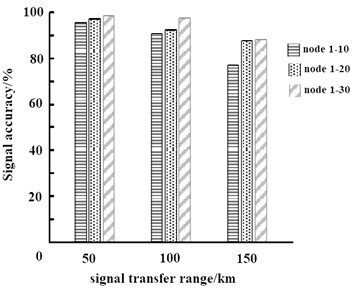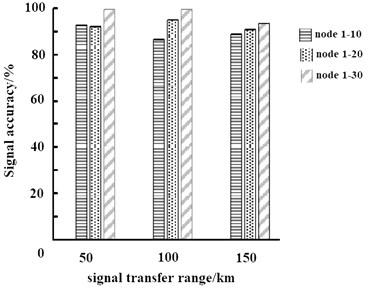b) Standard normalized precoding

## 5. Conclusions

The efficient and stable wireless transmission of data is the basis for the stable operation of the seismic monitoring system. Ensuring that the wireless network is in the best working state is one of the core elements of system design. Based on the 5G network framework, a wireless network structure with multi-point joint transmission characteristics can achieve good application results. Research shows that the optimal power control scheme is quite matched through numerical calculation. Compared with the traditional CB precoder, the CB precoder after normalization and normalization can provide greater gain, higher link rate, maximize the minimum rate of data transmission, and show good application effects under short-term power and long-term power conditions. The combination of high resolution and low resolution ADC can not only achieve high cost performance ratio, but also reduce the complexity and power consumption of system hardware. The influence of the number of network nodes on the spectrum efficiency and energy efficiency is monotonous, but for different quantization bits, the energy efficiency has a peak. Compared with Rayleigh channel, rice channel can support higher system and rate. In addition, an extremely low resolution ADC will greatly reduce the system performance, but the quantization distortion can be compensated by increasing the number of antennas at the AP end, and a 5-bit ADC can achieve a rate almost consistent with a perfect ADC. In addition, in the scenario of very strong Los components, the influence of low resolution ADC on channel estimation will disappear, but the negative effect on the uplink data detection stage still exists. The optimization of seismic signal wireless transmission system can effectively ensure the correctness and real-time of data, reduce power consumption and improve performance, which has a good role in promoting the technical development in the field of vibration detection.

09 November 2022
Accepted
27 February 2023
Published
01 August 2023
SUBJECTS
Seismic engineering and applications
Keywords
seismic monitoring system
wireless network
data transmission
energy efficiency
Acknowledgements

The paper is supported by provincial scientific research projects (62874155).

Data Availability

The datasets generated during and/or analyzed during the current study are available from the corresponding author on reasonable request.

Conflict of interest

The authors declare that they have no conflict of interest.